An antiderivative of a real function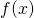defined on an interval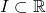is a function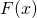whose derivative is equal to, that is,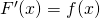, for all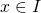. Antidifferentiation is the process of finding the set of all antiderivatives of a given function. Ifandare defined on the same interval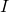, then the set of antiderivatives of the sum ofandequals the sum of the general antiderivatives ofand. In general, the antiderivatives of the product of two functionsanddo not coincide to the product of the antiderivatives ofand. Moreover, the fact thatandhave antiderivatives does not imply that the product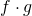has antiderivatives. Our aim in this paper is to present some conditions which ensure that the productand the composition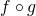of two functionsandhas antiderivatives.